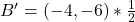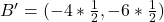## Triangle ABC has vertices located at A(1, -4), B(4, -6) and C(2, -1) on a coordinate grid. After it is reflected across the y-axis and a dil

Question

Triangle ABC has vertices located at A(1, -4), B(4, -6) and C(2, -1) on a coordinate grid. After it is reflected across the y-axis and a dilation of ½ is applied, what is the new coordinate of B? A. (4,6) B. (8,12) C. (2,3) D (-2, -3)

in progress 0
4 months 2021-08-06T09:22:21+00:00 1 Answers 5 views 0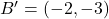Step-by-step explanation:

Given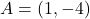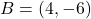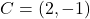reflection across y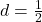Required

New coordinate of B

When reflected across the y-axis.

The rule is: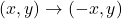So: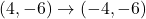Next is a dilation by 1/2

To do this, we multiply the new point by 1/2

So: